Anúncio

# 2_GLMs_printable.pdf

23 de Mar de 2023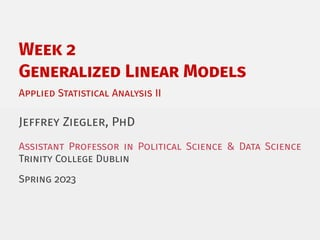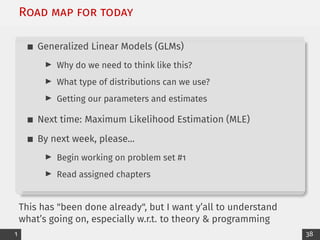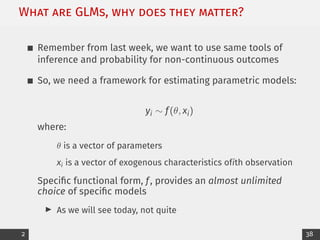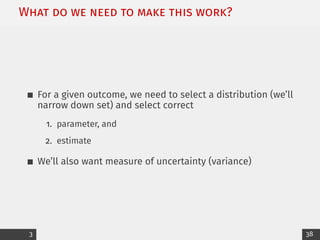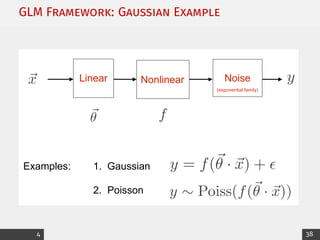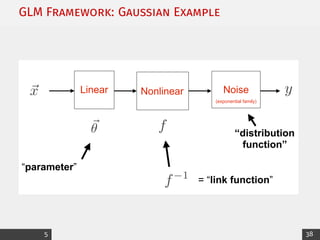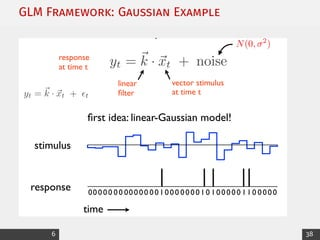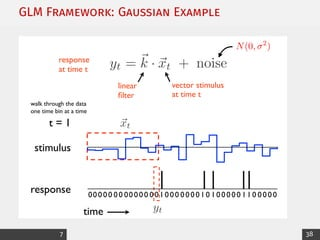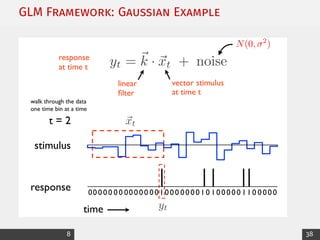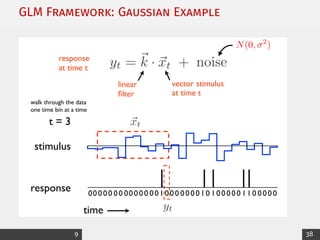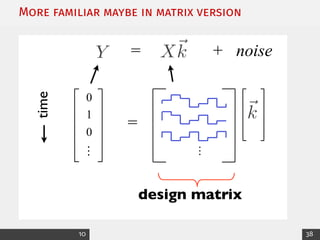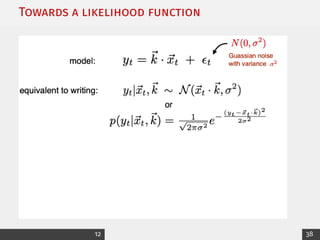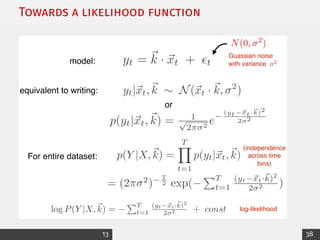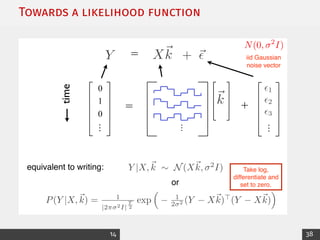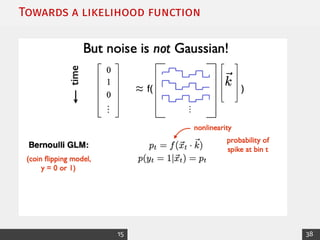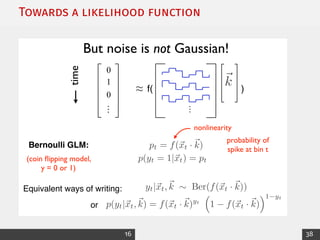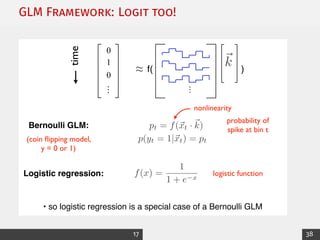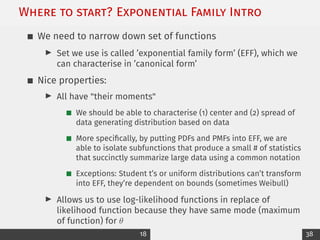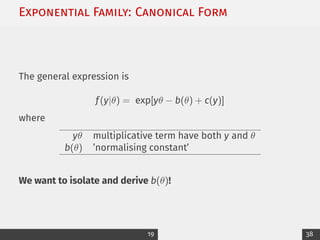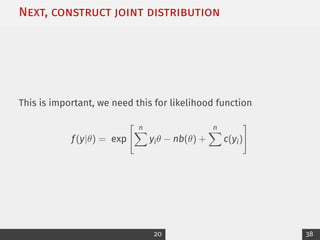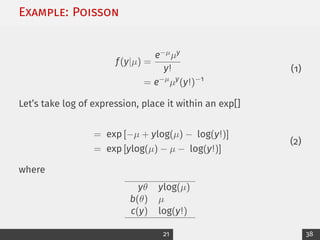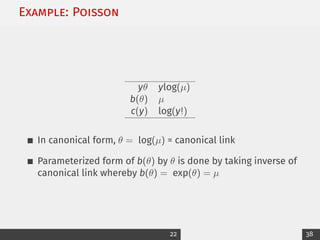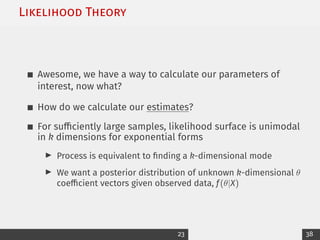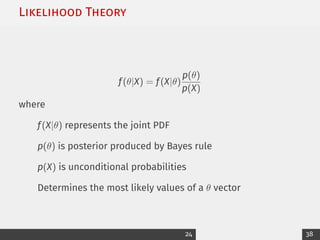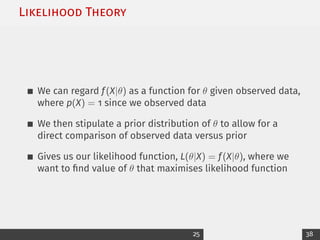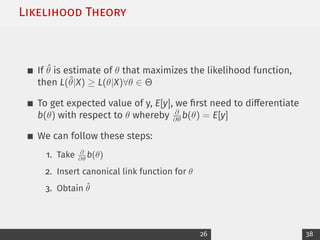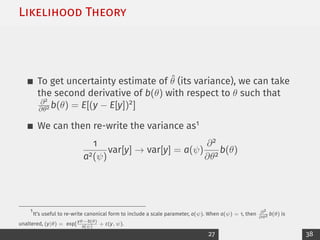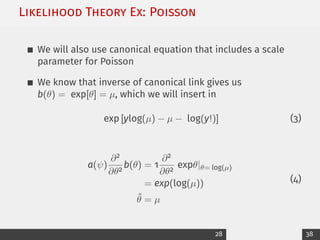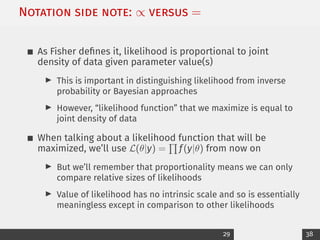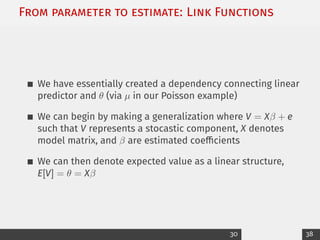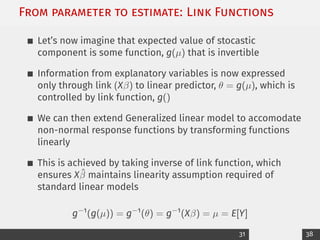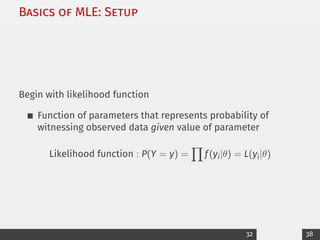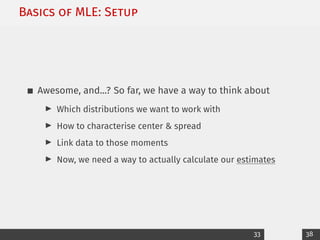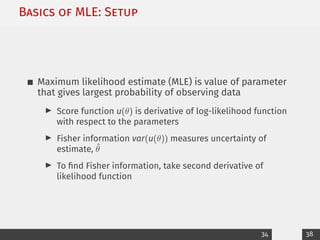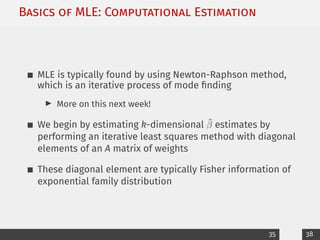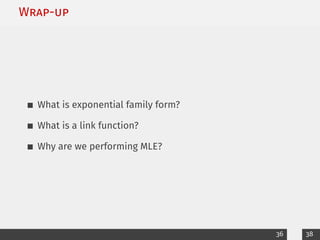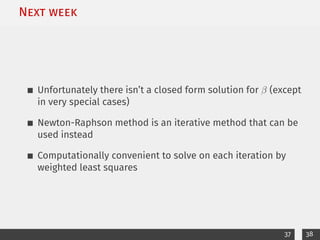1 de 39
Anúncio

### 2_GLMs_printable.pdf

1. Week 2 Generalized Linear Models Applied Statistical Analysis II Jeffrey Ziegler, PhD Assistant Professor in Political Science & Data Science Trinity College Dublin Spring 2023
2. Road map for today Generalized Linear Models (GLMs) I Why do we need to think like this? I What type of distributions can we use? I Getting our parameters and estimates Next time: Maximum Likelihood Estimation (MLE) By next week, please... I Begin working on problem set #1 I Read assigned chapters This has "been done already", but I want y’all to understand what’s going on, especially w.r.t. to theory & programming 1 38
3. What are GLMs, why does they matter? Remember from last week, we want to use same tools of inference and probability for non-continuous outcomes So, we need a framework for estimating parametric models: yi ∼ f(θ, xi) where: θ is a vector of parameters xi is a vector of exogenous characteristics ofith observation Specific functional form, f, provides an almost unlimited choice of specific models I As we will see today, not quite 2 38
4. What do we need to make this work? For a given outcome, we need to select a distribution (we’ll narrow down set) and select correct 1. parameter, and 2. estimate We’ll also want measure of uncertainty (variance) 3 38
5. GLM Framework: Gaussian Example 2. Generalized Linear Model Linear Examples: 1. Gaussian 2. Poisson Noise (exponential family) Nonlinear y = f(~ ✓ · ~ x) + ✏ 11 4 38
6. GLM Framework: Gaussian Example 2. Generalized Linear Model Linear Noise (exponential family) Nonlinear Terminology: “distribution function” “parameter” = “link function” 12 5 38
7. GLM Framework: Gaussian Example 0 0 1 0 0 0 1 0 0 0 0 0 0 1 0 1 01 0 0 0 00 0 0 0 0 0 0 0 0 0 0 0 0 0 0 stimulus response From s ike counts to spike trains: linear filter vector stimulus at time t time response at time t first idea: linear-Gaussian model! yt = ~ k · ~ xt + ✏t yt = ~ k · ~ xt + noise N(0, 2 ) 13 6 38
8. GLM Framework: Gaussian Example ~ xt yt 0 0 1 0 0 0 1 0 0 0 0 0 0 1 0 1 01 0 0 0 00 0 0 0 0 0 0 0 0 0 0 0 0 0 0 stimulus response linear filter vector stimulus at time t yt = ~ k · ~ xt + noise time response at time t t = 1 walk through the data one time bin at a time N(0, 2 ) 14 7 38
9. GLM Framework: Gaussian Example ~ xt yt 0 0 1 0 0 0 1 0 0 0 0 0 0 1 0 1 01 0 0 0 00 0 0 0 0 0 0 0 0 0 0 0 0 0 0 linear filter vector stimulus at time t yt = ~ k · ~ xt + noise time response at time t t = 2 walk through the data one time bin at a time stimulus response N(0, 2 ) 15 8 38
10. GLM Framework: Gaussian Example ~ xt yt 0 0 1 0 0 0 1 0 0 0 0 0 0 1 0 1 01 0 0 0 00 0 0 0 0 0 0 0 0 0 0 0 0 0 0 linear filter vector stimulus at time t yt = ~ k · ~ xt + noise time response at time t t = 3 walk through the data one time bin at a time stimulus response N(0, 2 ) 16 9 38
11. More familiar maybe in matrix version Build up to following matrix version: 0 … Y X~ k = + noise = time design matrix … ~ k 1 0 10 38
12. More familiar maybe in matrix version Build up to following matrix version: 0 1 0 … Y X~ k = + noise = time k̂ = (XT X) 1 XT Y stimulus covariance spike-triggered avg (STA) (maximum likelihood estimate for “Linear-Gaussian” GLM) least squares solution: … ~ k 21 11 38
13. Towards a likelihood function 12 38
14. Towards a likelihood function Formal treatment: scalar version model: N(0, 2 ) yt = ~ k · ~ xt + ✏t equivalent to writing: yt|~ xt,~ k ⇠ N(~ xt · ~ k, 2 ) p(yt|~ xt,~ k) = 1 p 2⇡ 2 e (yt ~ xt·~ k)2 2 2 or p(Y |X,~ k) = T Y t=1 p(yt|~ xt,~ k) For entire dataset: (independence across time bins) = (2⇡ 2 ) T 2 exp( PT t=1 (yt ~ xt·~ k)2 2 2 ) Guassian noise with variance 2 log P(Y |X,~ k) = PT t=1 (yt ~ xt·~ k)2 2 2 + const log-likelihood 22 13 38
15. Towards a likelihood function Formal treatment: vector version 0 … Y X~ k = … ~ k = time + ~ ✏ N(0, 2 I) … + iid Gaussian noise vector ✏1 ✏2 ✏3 equivalent to writing: or Y |X,~ k ⇠ N(X~ k, 2 I) P(Y |X,~ k) = 1 |2⇡ 2I| T 2 exp ⇣ 1 2 2 (Y X~ k)> (Y X~ k) ⌘ Take log, differentiate and set to zero. 1 0 23 14 38
16. Towards a likelihood function 15 38
17. Towards a likelihood function 0 … … ~ k ≈ time probability of spike at bin t Bernoulli GLM: pt = f(~ xt · ~ k) (coin flipping model, y = 0 or 1) p(yt = 1|~ xt) = pt nonlinearity Equivalent ways of writing: yt|~ xt,~ k ⇠ Ber(f(~ xt · ~ k)) p(yt|~ xt,~ k) = f(~ xt · ~ k)yt ⇣ 1 f(~ xt · ~ k) ⌘1 yt or But noise is not Gaussian! log-likelihood: L = PT t=1 ⇣ yt log f(~ xt · ~ k) + (1 yt) log(1 f(~ xt · ~ k)) ⌘ f( ) 1 0 16 38
18. GLM Framework: Logit too! Logistic regression Logistic regression: f(x) = 1 1 + e x logistic function • so logistic regression is a special case of a Bernoulli GLM 0 … … ~ k ≈ time probability of spike at bin t Bernoulli GLM: pt = f(~ xt · ~ k) (coin flipping model, y = 0 or 1) p(yt = 1|~ xt) = pt nonlinearity f( ) 1 0 25 17 38
19. Where to start? Exponential Family Intro We need to narrow down set of functions I Set we use is called ’exponential family form’ (EFF), which we can characterise in ’canonical form’ Nice properties: I All have "their moments" We should be able to characterise (1) center and (2) spread of data generating distribution based on data More specifically, by putting PDFs and PMFs into EFF, we are able to isolate subfunctions that produce a small # of statistics that succinctly summarize large data using a common notation Exceptions: Student t’s or uniform distributions can’t transform into EFF, they’re dependent on bounds (sometimes Weibull) I Allows us to use log-likelihood functions in replace of likelihood function because they have same mode (maximum of function) for θ 18 38
20. Exponential Family: Canonical Form The general expression is f(y|θ) = exp[yθ − b(θ) + c(y)] where yθ multiplicative term have both y and θ b(θ) ’normalising constant’ We want to isolate and derive b(θ)! 19 38
21. Next, construct joint distribution This is important, we need this for likelihood function f(y|θ) = exp " n X yiθ − nb(θ) + n X c(yi) # 20 38
22. Example: Poisson f(y|µ) = e−µµy y! = e−µ µy (y!)−1 (1) Let’s take log of expression, place it within an exp[] = exp [−µ + ylog(µ) − log(y!)] = exp [ylog(µ) − µ − log(y!)] (2) where yθ ylog(µ) b(θ) µ c(y) log(y!) 21 38
23. Example: Poisson yθ ylog(µ) b(θ) µ c(y) log(y!) In canonical form, θ = log(µ) = canonical link Parameterized form of b(θ) by θ is done by taking inverse of canonical link whereby b(θ) = exp(θ) = µ 22 38
24. Likelihood Theory Awesome, we have a way to calculate our parameters of interest, now what? How do we calculate our estimates? For sufficiently large samples, likelihood surface is unimodal in k dimensions for exponential forms I Process is equivalent to finding a k-dimensional mode I We want a posterior distribution of unknown k-dimensional θ coefficient vectors given observed data, f(θ|X) 23 38
25. Likelihood Theory f(θ|X) = f(X|θ) p(θ) p(X) where f(X|θ) represents the joint PDF p(θ) is posterior produced by Bayes rule p(X) is unconditional probabilities Determines the most likely values of a θ vector 24 38
26. Likelihood Theory We can regard f(X|θ) as a function for θ given observed data, where p(X) = 1 since we observed data We then stipulate a prior distribution of θ to allow for a direct comparison of observed data versus prior Gives us our likelihood function, L(θ|X) = f(X|θ), where we want to find value of θ that maximises likelihood function 25 38
27. Likelihood Theory If θ̂ is estimate of θ that maximizes the likelihood function, then L(θ̂|X) ≥ L(θ|X)∀θ ∈ Θ To get expected value of y, E[y], we first need to differentiate b(θ) with respect to θ whereby ∂ ∂θ b(θ) = E[y] We can follow these steps: 1. Take ∂ ∂θ b(θ) 2. Insert canonical link function for θ 3. Obtain θ̂ 26 38
28. Likelihood Theory To get uncertainty estimate of θ̂ (its variance), we can take the second derivative of b(θ) with respect to θ such that ∂2 ∂θ2 b(θ) = E[(y − E[y])2] We can then re-write the variance as1 1 a2(ψ) var[y] → var[y] = a(ψ) ∂2 ∂θ2 b(θ) 1 It’s useful to re-write canonical form to include a scale parameter, a(ψ). When a(ψ) = 1, then ∂2 ∂θ2 b(θ) is unaltered, (y|θ) = exp[ yθ−b(θ) a(ψ) + c(y, ψ). 27 38
29. Likelihood Theory Ex: Poisson We will also use canonical equation that includes a scale parameter for Poisson We know that inverse of canonical link gives us b(θ) = exp[θ] = µ, which we will insert in exp [ylog(µ) − µ − log(y!)] (3) a(ψ) ∂2 ∂θ2 b(θ) = 1 ∂2 ∂θ2 expθ|θ= log(µ) = exp(log(µ)) θ̂ = µ (4) 28 38
30. Notation side note: ∝ versus = As Fisher defines it, likelihood is proportional to joint density of data given parameter value(s) I This is important in distinguishing likelihood from inverse probability or Bayesian approaches I However, “likelihood function” that we maximize is equal to joint density of data When talking about a likelihood function that will be maximized, we’ll use L(θ|y) = Q f(y|θ) from now on I But we’ll remember that proportionality means we can only compare relative sizes of likelihoods I Value of likelihood has no intrinsic scale and so is essentially meaningless except in comparison to other likelihoods 29 38
31. From parameter to estimate: Link Functions We have essentially created a dependency connecting linear predictor and θ (via µ in our Poisson example) We can begin by making a generalization where V = Xβ + e such that V represents a stocastic component, X denotes model matrix, and β are estimated coefficients We can then denote expected value as a linear structure, E[V] = θ = Xβ 30 38
32. From parameter to estimate: Link Functions Let’s now imagine that expected value of stocastic component is some function, g(µ) that is invertible Information from explanatory variables is now expressed only through link (Xβ) to linear predictor, θ = g(µ), which is controlled by link function, g() We can then extend Generalized linear model to accomodate non-normal response functions by transforming functions linearly This is achieved by taking inverse of link function, which ensures Xβ̂ maintains linearity assumption required of standard linear models g−1 (g(µ)) = g−1 (θ) = g−1 (Xβ) = µ = E[Y] 31 38
33. Basics of MLE: Setup Begin with likelihood function Function of parameters that represents probability of witnessing observed data given value of parameter Likelihood function : P(Y = y) = Y f(yi|θ) = L(yi|θ) 32 38
34. Basics of MLE: Setup Awesome, and...? So far, we have a way to think about I Which distributions we want to work with I How to characterise center & spread I Link data to those moments I Now, we need a way to actually calculate our estimates 33 38
35. Basics of MLE: Setup Maximum likelihood estimate (MLE) is value of parameter that gives largest probability of observing data I Score function u(θ) is derivative of log-likelihood function with respect to the parameters I Fisher information var(u(θ)) measures uncertainty of estimate, θ̂ I To find Fisher information, take second derivative of likelihood function 34 38
36. Basics of MLE: Computational Estimation MLE is typically found by using Newton-Raphson method, which is an iterative process of mode finding I More on this next week! We begin by estimating k-dimensional β̂ estimates by performing an iterative least squares method with diagonal elements of an A matrix of weights These diagonal element are typically Fisher information of exponential family distribution 35 38
37. Wrap-up What is exponential family form? What is a link function? Why are we performing MLE? 36 38
38. Next week Unfortunately there isn’t a closed form solution for β (except in very special cases) Newton-Raphson method is an iterative method that can be used instead Computationally convenient to solve on each iteration by weighted least squares 37 38
39. Class business Read required (and suggested) online materials Problem set # 1 is up on GitHub Next time, we’ll talk about how to actually maximise our likelihood functions ! 38 / 38
Anúncio